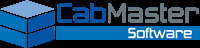﻿ Examples, Hints And TipsIn This Topic
Examples, Hints And Tips
In This Topic

## Layer Names

### Why Are There Quotes Around Most Layer Names?

The quotes around the Names stored in the Layers Table are required to cater for the use of Formulas (see below) in the name. In CabMaster terms this makes each entry a formula even if it is just a fixed name.

### Creating Multiple Strategies From A Single Layer

If a single layer from CabMaster needs to be translated into multiple tool strategies (for example, a 2 tool strategy to route out a part), then enter the layer names into the Layers Table delimited by the pipe character ( | ).

For example, "Border1|Border2".

### Using A Formula to Control A Layer Name

If a Layer Name needs to vary according to a property of the material being machined (for example, its Thickness or Raw Material), then the formula can be entered in place of the Layer Name.

A formula does not require quotes around it, but fixed textural values within the formula do.

Example 1 :

"Border-"&Material

will produce the Raw Material (from the part's material definition) appended to the text "Border-", e.g.

Border-HMR

Example 2 :

"Border1|Border2-"&Dim3

will produce two layers.  The first, simply

Border

the second, being the Thickness (from the part's material definition) appended to the text "Border2-", e.g.

Border2-16mm

An understanding of the CabMaster formula language and internal property names is required to be fully proficient in writing a formula.  However, the structure of simple formulas is quite straight forward.  Please call CabMaster Support for assistance.

Note : There needs to be an associated entry in the 'Override' table to map each possible Layer name to a Strategy.  If one does not exist the 'Default' entry in the Override table is used.

## Strategies

### What is a Strategy Evaluation Formula?

Strategy evaluation formulas allow the defined Strategy Properties and other information to be combined into a useable Strategy.  They are formulas written in the CabMaster formula language and can be reasonably complex.  However, the default examples provided can be used as templates to create new ones.

Example formula (colour coded for ease of reference) :

let Me:="RouteMale1";

let MyInfo:=MachToolLayerTableStrategyInfo(Me);
let MyInfoIndex:=
MachToolLayerTableStrategyIndex(Me);

let MyDepth:=TextNoUnit(MRound(Depth.x*100));

let MyOperation:=MyInfo(IndexOf("Operation", MyInfoIndex));

"TCHW0B"& MyOperation &"D"& MyDepth

This formula can be broken down into 5 different elements:

• Defining 'Me'

Each formula should start with the setting of the local variable 'Me' to the exact name of the saved Strategy itself.

• Defining 'MyInfo' and 'MyInfoIndex'

These lines define two fixed local variables, needed if any of the Strategy's saved Property values are to be referenced. Their definition will always be the same and so do not need to be edited.

• Defining 'Special' variables

These lines define any special local values (in this example the plunge Depth is multiplied by 100 and rounded to the nearest whole number and then the units [i.e. 'mm'] removed.  In this case, with a depth of 16.004mm, MyDepth would evaluate to '1600').

• Defining 'Property' variables

These lines extract the Strategy's saved Property values. Each line is always be of the same format with the text "Operation" (shown above) replaced with the required property name.

• Output

This is the part of the formula that defines how the resulting strategy will read. It can be a mixture of "Text", Special variables, Standard variables and other formulas.  Concatenate the different elements together by using the '&' character.

e.g. using the sample formula above:

• With
• the property "Operation" defined with a value of '8'
• a CabMaster machining "Depth" of '16.004mm'
• Then the resulting strategy would be
•  TCHW0B8D1600

An understanding of the CabMaster formula language and internal property names is required to be fully proficient in writing a formula, even though the structure of simple formulas is quite straight forward.

Please contact CabMaster Support for assistance.

## Useful CabMaster Properties

The following are useful property names that can be included in formulas

• Category = The current part's usage (e.g. Carcass, Door, Panel etc)
• Material = The current part's Raw Material name (as defined in the Materials table)
• Dim1 = The current part's height (or depth if horizontal) - including edging
• DimCut1 = The current part's height (or depth if horizontal) - not including edging
• Dim2 = The current part's width - including edging
• DimCut2 = The current part's width - not including edging
• Dim3 = The current part's Thickness
• Depth = Depth of the defined machining